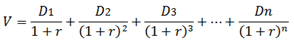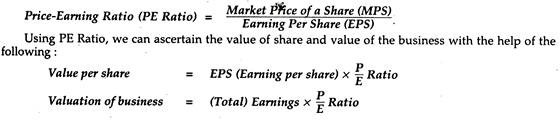# Valuation of Securities: Debentures, Preference Shares, and Equity Shares

### Valuation of Convertible Bonds/Debentures

Debentures enjoy the benefits of both debt (initially) and equity (later). The terms of conversion will decide the pricing of these convertibles, which is left to free market forces in India, now. It is the company’s perception what they can sell to investors and at what price?

As per SEBI guidelines, Fully Convertible Debentures (FCDs) and Partly Convertible Bonds (PCBs) have special features and are not governed by guidelines for Non-Convertible Bonds/Debentures (NCDs). Their pricing methods are differ­ent from bonds.

Why Convertible Financing?

(1) It will delay the issue of equity and hence dilution of earnings per share is delayed.

(2) It will give breathing time for the company for increasing its earnings, when it will be in a position to expand equity base and service them.

(3) Initial bond financing will earn leverage for the company as interest expense on debt is not taxable and the cost of debt financing is lower than that of equity for some companies.

(4) Companies in initial stages of expansion or project financing, or with low credit rating but with the potential for larger earnings in a couple of years prefer convertibles.

(5) Investors are attracted to convertibles, due to the sweeteners like premium on conversion and dividends and capital appreciation later.

VALUATION OF PREFERENCE SHARES

Preferred shares have the qualities of stocks and bonds, which makes their valuation a little different than that of common shares. The owners of preferred shares are part owners of the company in proportion to the held stocks, just like common shareholders.

If preferred stocks have a fixed dividend, then we can calculate the value by discounting each of these payments to the present day. This fixed dividend is not guaranteed in common shares. If you take these payments and calculate the sum of the present values into perpetuity, you will find the value of the stock.

For example, if ABC Company pays a 25-cent dividend every month and the required rate of return is 6% per year, then the expected value of the stock, using the dividend discount approach, would be \$50. The discount rate was divided by 12 to get 0.005, but you could also use the yearly dividend of \$3 (0.25 x 12) and divide it by the yearly discount rate of 0.06 to get \$50. In other words, you need to discount each dividend payment that’s issued in the future back to the present, then add each value together.Where:

V = the value

D1 = the dividend next period

r = the required rate of return

### VALUATION OF EQUITY SHARES

Equity valuation methods can be broadly classified into balance sheet methods, discounted cash flow methods, and relative valuation methods. Balance sheet methods comprise of book value, liquidation value, and replacement value methods. Discounted cash flow methods include dividend discount models and free cash flow models.  Lastly, relative valuation methods are a price to earnings ratios, price to book value ratios, price to sales ratios etc

Methods of Valuation of Shares

1. Asset-Backing Method

Since the valuation is made on the basis of the assets of the company, it is known as Asset-Basis or Asset- Backing Method. At the same time, the shares are valued on the basis of real internal value of the assets of the company and that is why the method is also termed Intrinsic Value Method or Real Value Basis Method.

This method may be made either

(i) On a going/continuing concern basis; and

(ii) Break-up value basis.

1. Yield-Basis Method

Yield is the effective rate of return on investments which is invested by the investors. It is always expressed in terms of percentage. Since the valuation of shares is made on the basis of Yield, it is called Yield-Basis Method. For example, an investor purchases one share of Rs. 100 (face value and paid-up value) at Rs. 150 from a Stock Exchange on which he receives a return (dividend) @ 20%.

Under Yield-Basis method, valuation of shares is made on;

(i) Profit Basis

(ii) Dividend Basis.

1. Fair Value Method

There are some accountants who do not prefer to use Intrinsic Value or Yield Value for ascertaining the correct value of shares. They, however, prescribe the Fair Value Method which is the mean of Intrinsic Value Method end Yield Value Method. The same provides a better indication about the value of shares than the earlier two methods.1. Return on Capital Employed Method

Under this method, valuation of share is made on the basis of rate of a return (after tax) on capital employed. Rates of return are taken on the basis of predetermined/expected rates of return which an investor may expect on the investments. After ascertaining this expected earnings, we are to determine the capital sum for such a return.

1. Price-Earnings Ratio Method

We know that it is the ratio which relates the market price of the share to earning per equity share.

It is calculated as:## 4 thoughts on “Valuation of Securities: Debentures, Preference Shares, and Equity Shares”

error: Content is protected !!